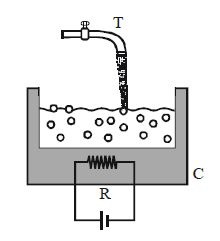# Water Level remains constant!

Water at temperature $40°C$ flows from a tap $T$ into a heated container $C$. The container has a heating element (a resistor $R$) which is generating heat at the rate of $P$, that may be varied. The rate of water in flow from tap is $m = \frac{1000}{7} L/min$.

The heat generated is sufficient so that the water in the container is boiling and getting converted into steam at a steady rate. What is the power $P$ (in $MW$) that must be generated as heat in the steady state in resistor $R$ so that the amount of liquid water in the container neither increases nor decreases with time ?

(Neglect other losses of heat, such as conduction from the container to the air and heat capacity of container)

Note - For water, specific heat $c = 4.2 kJ kg^{-1} K^{-1}$, latent heat of vaporization $L_{vap} = 2.268 MJ kg^{-1}$, density $\rho = 1000 kg m^{-3}$.×

Problem Loading...

Note Loading...

Set Loading...